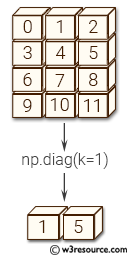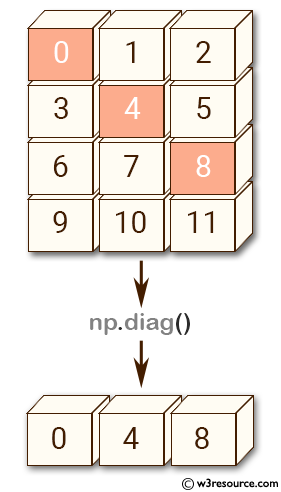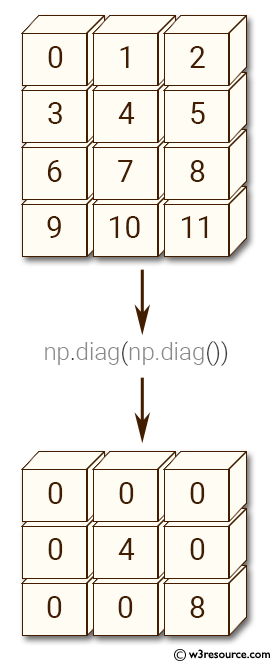# NumPy array creation: diag() function

## numpy.diag() function

The diag() function is used to extract a diagonal or construct a diagonal array.

Syntax:

`numpy.diag(v, k=0)`Version: 1.15.0

Parameter:

Name Description Required /
Optional
v If v is a 2-D array, return a copy of its k-th diagonal. If v is a 1-D array, return a 2-D array with v on the k-th diagonal. Required
k Diagonal in question. The default is 0. Use k>0 for diagonals above the main diagonal, and k<0 for diagonals below the main diagonal. optional

Return value:

out : ndarray - The extracted diagonal or constructed diagonal array.

Example-1: NumPy.diag() function

``````>>> import numpy as np
>>> a = np.arange(12).reshape((4,3))
>>> np.diag(a)
array([0, 4, 8])
``````

Pictorial Presentation:Example-2: NumPy.diag() function

``````>>> import numpy as np
>>> a = np.arange(12).reshape((4,3))
>>> np.diag(a, k=1)
array([1, 5])
``````

Example-3: NumPy.diag() function

``````>>> import numpy as np
>>> a = np.arange(12).reshape((4,3))
>>> np.diag(a, k=-1)
array([ 3,  7, 11])
``````

Example-4: NumPy.diag() function

``````>>> import numpy as np
>>> a = np.arange(12).reshape((4,3))
>>> np.diag(np.diag(a))
array([[0, 0, 0],
[0, 4, 0],
[0, 0, 8]])
``````

Pictorial Presentation:Python - NumPy Code Editor:

Previous: ogrid()
Next: diagflat()

﻿